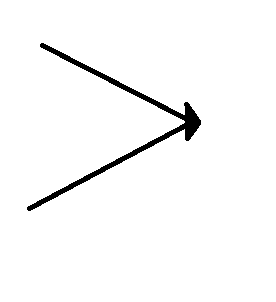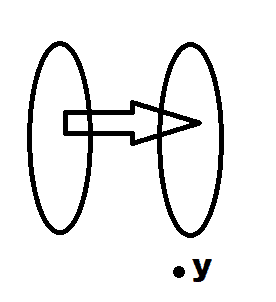# Finding a useful denial of a injective function and a surjective function

• ver_mathstats

## Homework Statement

Find the useful denial of a injective function and a surjective function.

## The Attempt at a Solution

I know a one to one function is (∀x1,x2 ∈ X)(x1≠x2 ⇒ f(x1) ≠ f(x2)). So would the useful denial be (∃x1,x2 ∈ X)(x1 ≠ x2 ∧ f(x1) = f(x2))?

I know a onto function is (∀y ∈ Y)(∃x ∈ X)(y=f(x)). So would the useful denial be (∃y ∈ Y)(∀x ∈ X)(y≠f(x))?

Thank you.

## Homework Statement

Find the useful denial of a injective function and a surjective function.

## The Attempt at a Solution

I know a one to one function is (∀x1,x2 ∈ X)(x1≠x2 ⇒ f(x1) ≠ f(x2)). So would the useful denial be (∃x1,x2 ∈ X)(x1 ≠ x2 ∧ f(x1) = f(x2))?

I know a onto function is (∀y ∈ Y)(∃x ∈ X)(y=f(x)). So would the useful denial be (∃y ∈ Y)(∀x ∈ X)(y≠f(x))?

Thank you.
Both is correct.

Both is correct.
Thank you.

Injective meanscannot happen, and surjective means##y## cannot exist.